# Hough

The Hough transform is a technique for creating lines based on points. The results of a typical edge detection routine are many unconnected points. To us it is obvious that these points represent shapes but because the points are not connected it is difficult for a machine to understand the underlying shape. The Hough transform takes as input many points and will generate guesses for what lines those points represent.

The Hough module interface shows the parameter space image that represents the Hough transform in a visual manner. The image axis are the angular amount (theta) and the distance value (r) used to represent a line using polar notation "r = x cos(theta) + y sin(theta)" as apposed to coordinate notation "y = m x + b". The Hough transform converts potential lines into peaks within this image. The task of detecting lines now instead becomes a task of detecting peaks within this image. The interface below shows green squares around those detected peaks which indicate the parameters (theta,r) of the lines seen in the below example. By thresholding and isolating these peaks it is possible to get a reasonably good approximation of the actual figure (source image -> Hough transform).

For a detailed description of how the Hough transform works see the links below.

## Interface## Instructions

1. Setup - To use the Hough transform it is typical to first use an edge detection routine like the Sobel Edge. This helps identify points to use in the transform and can speed up processing by eliminating points (i.e. setting them to black) that do not need to be processed.

2. Threshold - Increase or decrease the "Threshold" number field or scroll the horizontal scroller until a number of lines appear in the image.

3. Isolation - Often many lines that are detected are very close in slope and proximity to each other and can create a meaningless image. Use the peak isolation number to remove lines whose slopes are close to each other. See below for a visual example.

4. Sharpness - Not all detected Hough Peaks are very sharp which produce lines which are created from noise within the image. Increase the sharpness value until lines that do not have sharp support are removed from the display. These errors happen often when the prior edge detection module creates a high response in a flat area due to texture noise. As that is not a true edge the resulting peak's shape will not be sharp.

5. Peak Count - If you know how many lines you wish to detect enter that number in the Peak Count textbox. This will ensure that the module selects only the X highest peaks/lines as a result of the hough transform. This can be more adaptive than setting the threshold as that can change based on image size, edge detection method used, etc.

6. Precision - If the module causes your machine to slow down or hang use the "Precision" to reduce the computational load on your machine. The Hough transform is VERY cpu intensive and not all the points need to be analyzed in order for a useful result to appear. Changing the "Precision" value will reduce the number of points used in the transform by skipping over X number of points.

7. Edge Graident - If you have a gradient image (as apposed to a binary image) then checking the gradient will cause the transform to take into account the intensity of the edge. Thus strong edges will result in a higher peak value than weak edges which can make threshold detection easier. Note that this disables the bounding of lines.

8. Bound Lines - Select the bound lines checkbox if you want to bound each line to those points that define the extreme points of the detected line. (Black/White Images only)

9. Min/Max Angle Filter - Specify the line angles that you want to ignore from the results. Note that the range is 0 to 180 degrees with 0 and 180 being horizontal lines and 90 being vertical. Thus if you wanted to eliminate horizontal lines you could use 20 and 160 as the range. If you wanted to eliminate vertical lines then using 100 and 70 as the range would remove angles 70 to 100. Note that if min is larger than max, the longer arc (i.e. from 100 to 180 and then 0 to 70 would be in range) is used due to circular math.

10. Line Color - The color of the detected lines drawn in the main image (and also the peak indicators).

11. Line Thickness - The thickness to use when drawing the detected lines in the main image.

12. Overlay On - Select if you want to draw the detected lines ontop of your source image to help you understand why lines are appearing.

## Example

 Source Hough LinesHough Lines with peak isolation of 1 Bounded Hough Lines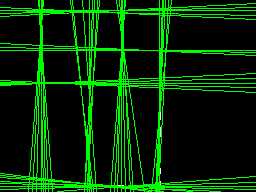## Variables

HOUGH_LINES - contains the lines found from the Hough transform. Using code similar to the following within a VBScript module will allow you to further process the line segments.

HOUGH_LINE_INTENSITIES - contains the strength or height values for the lines found from Hough transform. Using these codes you can determine a level of confidence associated with the line. Note that this array is 4x smaller than the HOUGH_LINES array.

```    line = GetArrayVariable("HOUGH_LINES")
inten = GetArrayVariable("HOUGH_LINE_INTENSITIES")

' write back to messages the first line ..
' note that line segments are defined by two endpoints
Write("First Coord: " & line(0) & "," & line(1) & vbCRLF)
Write("Second Coord: " & line(2) & "," & line(3))
Write("Strength: " & inten(0))
```

 Hough Related Forum Posts Last post Posts Views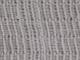HELP - how to detect and draw lines in RoboRealm Hy! I have a big problem :) I need the program to draw me lines like i... 10 year 2 2712 Hough Transform - Angles I've read other posts about Hough angle limits but I am still confused.  The documentation for the module does not de... 10 year 2 2185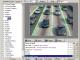lane detecting Please Any can suggest me to do the lane detection like the picture which I attached. and How can I detect the broken line in th... 13 year 2 3837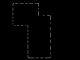Bug in new Angle Filter in Hough Module? Hello, I was trying out the new Angle Filter function in the Hough Transform module and either I do... 13 year 3 3495Roborealm to read an analog meter STeven,   It was great talking with you at the MiniMaker Fair yesterday!  Here are some sample... 13 year 3 3427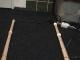Outdoor navigation Hello everyone I'am Bob. I'am part of a project group at my school and we are building a robot which should be a... 14 year 7 3734